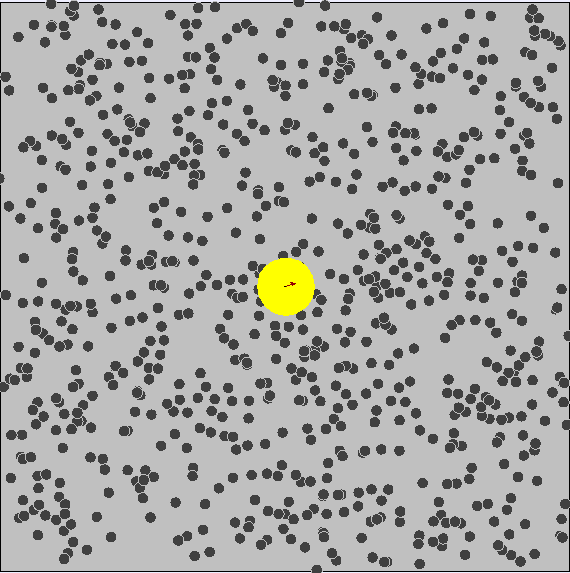# Forces Acting on Colloids¶

There are four forces that act on colloidal particles in suspension:

1. Gravitational force - Settles or raises the particles based on their relative density
2. Viscous drag force - How much the particles resist gravity based on its interaction with the solvent (think cavitation)
3. Kinetic energy of particles - Brownian motion
4. Intermolecular forces

## Gravitational and Viscous Forces¶

We can calculate the terminal velocity of a spherical particle going through water.

The two forces must be balanced for the particles to stay in suspension

\begin{align}\text{viscous drag}&=\text{gravity}\\ 6\pi rV\frac{\eta}{1000}&=\frac{4}{3}\pi r^3g\big(\rho_p-\rho_w\big) \end{align}
• $$r=$$ radius ($$m$$)
• $$V=$$ velocity ($$m\cdot s^{-1}$$)
• $$\eta=$$ viscocity ($$0.8904\:cP$$ - $$(kg·(m·s)^{-1})$$)
• $$g=$$ acceleration due to gravity ($$9.81\:m\cdot s^{-1}$$)
• $$\rho=$$density ($$kg\cdot m^{-3}$$)

Example 1 - Calculate the terminal velocity of a spherical particle in water with a density of $$2\:g/mL$$ and a particle radius of $$100\:\mu m$$

\begin{align} 6\pi rV\frac{\eta}{1000}&=\frac{4}{3}\pi r^3g\big(\rho_p-\rho_w\big)\\ V&=\frac{4000\pi r^3g\big(\rho_p-\rho_w\big)}{18 \pi r\eta}\\ V&=\frac{4000\bcancel{\pi} r^\bcancel{3}g\big(\rho_p-\rho_w\big)}{18 \bcancel{\pi} \bcancel{r}\eta}\\ V&=\frac{4000 r^2g\big(\rho_p-\rho_w\big)}{18\eta}\\ V&=\frac{4000 (100\times10^{-6}\:m)^2(9.81m\cdot s^{-1})\big((2000\:kg\cdot m^{-3})-(1000\:kg\cdot m^{-3})\big)}{18(0.8904\:kg·m−1·s−1)}\\ V&=\frac{0.3924}{16.0272}\\ V&=0.024\:m/s \end{align}

$$100\:\mu m$$ is massive though, so if we had a much smaller particle, say $$r=10\:nm$$, we’d end up with:

\begin{align} V&=\frac{4000 (10\times10^{-9}\:m)^2(9.81m\cdot s^{-1})\big((2000\:kg\cdot m^{-3})-(1000\:kg\cdot m^{-3})\big)}{18(0.8904\:kg·m−1·s−1)}\\ V&=\frac{3.924\times10^{-9}}{16.0272}\\ V&=2.45\times10^{-10}\:m/s \end{align}

It would take 13.2 years for that particle to move $$10\:cm$$

## Brownian Motion¶

We know that particles have kinetic energy, which makes them move around and bump into each other. This is called brownian motion.

We can use the kinetic energy equation to determine how fast this particle will be moving

$\frac{1}{2}mv^2=kT$
• $$m=$$ mass ($$kg$$)
• $$v=$$ velocity ($$m\cdot s^{-1}$$)
• $$k=$$ Boltzmann constant ($$1.381\times 10^{-23}\:J\cdot K^{-1}$$)
• $$T=$$ temperature ($$K$$)

Example 2 - Calculate the velocity of a particle with density of $$2\:g/mL$$ and a radius of $$100\:\mu m$$ at $$25^\circ C$$

\begin{align} \frac{1}{2}mv^2&=kT\\ v&=\sqrt{\frac{2kT}{m}}\\ v&=\sqrt{\frac{2kT}{(\rho=\frac{m}{v})}}\\ v&=\sqrt{\frac{2kT}{(\rho v=m)}}\\ v&=\sqrt{\frac{2kT}{\rho v}}\\ v&=\sqrt{\frac{2kT}{\rho(\frac{4}{3}\pi r^3)}}\\ v&=\sqrt{\frac{2(1.381\times 10^{-23}\:J\cdot K^{-1})(298.15\:K)}{2000\:kg\cdot m^{-3}(\frac{4}{3}\pi (100\times10^{-6}\:m)^3)}}\\ v&=9.92\times10^{-7}\:m\cdot s^{-1} \end{align}

At $$r=10\:nm$$ we get:

\begin{align} v&=\sqrt{\frac{2(1.381\times 10^{-23}\:J\cdot K^{-1})(298.15\:K)}{2000\:kg\cdot m^{-3}(\frac{4}{3}\pi (10\times10^{-9}\:m)^3)}}\\ v&=0.99\:m\cdot s^{-1} \end{align}

As a result, small particles have much more kinetic energy and collisions occur much more often

• If there are attractive intermolecular forces, these may result in further aggregation
• In increase in size results in an increase in gravitational velocity and thus increase in colloidal settling